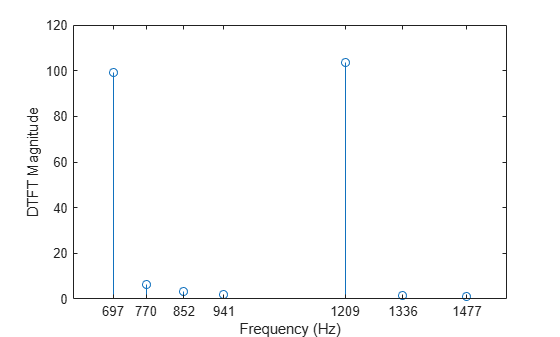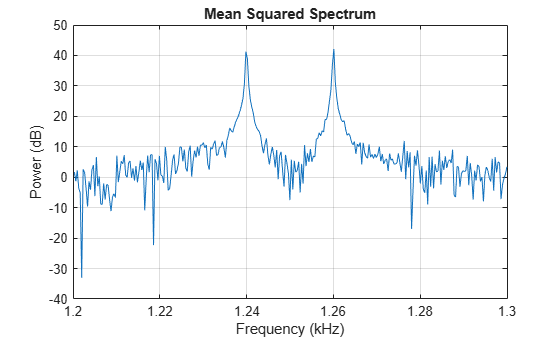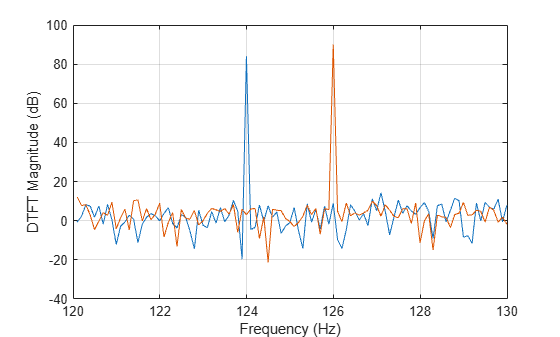# goertzel

Discrete Fourier transform with second-order Goertzel algorithm

## Syntax

``dft = goertzel(data)``
``dft = goertzel(data,findx)``
``dft = goertzel(data,findx,dim)``

## Description

example

````dft = goertzel(data)` returns the discrete Fourier transform (DFT) of the input array `data` using a second-order Goertzel algorithm. If `data` has more than one dimension, then `goertzel` operates along the first array dimension with size greater than 1.```

example

````dft = goertzel(data,findx)` returns the DFT for the frequency indices specified in `findx`.```

example

````dft = goertzel(data,findx,dim)` computes the DFT along dimension `dim`. To input a dimension and use the default value of `findx`, specify the second argument as empty, `[]`.```

## Examples

collapse all

Estimate the frequencies of the tone generated by pressing the 1 button on a telephone keypad.

The number 1 produces a tone with frequencies 697 and 1209 Hz. Generate 205 samples of the tone with a sample rate of 8 kHz.

```Fs = 8000; N = 205; lo = sin(2*pi*697*(0:N-1)/Fs); hi = sin(2*pi*1209*(0:N-1)/Fs); data = lo + hi;```

Use the Goertzel algorithm to compute the discrete Fourier transform (DFT) of the tone. Choose the indices corresponding to the frequencies used to generate the numbers 0 through 9.

```f = [697 770 852 941 1209 1336 1477]; freq_indices = round(f/Fs*N) + 1; dft_data = goertzel(data,freq_indices);```

Plot the DFT magnitude.

```stem(f,abs(dft_data)) ax = gca; ax.XTick = f; xlabel('Frequency (Hz)') ylabel('DFT Magnitude')```Generate a noisy cosine with frequency components at 1.24 kHz, 1.26 kHz, and 10 kHz. Specify a sample rate of 32 kHz. Reset the random number generator for reproducible results.

```rng default Fs = 32e3; t = 0:1/Fs:2.96; x = cos(2*pi*t*10e3) + cos(2*pi*t*1.24e3) + cos(2*pi*t*1.26e3) ... + randn(size(t));```

Generate the frequency vector. Use the Goertzel algorithm to compute the DFT. Restrict the range of frequencies to between 1.2 and 1.3 kHz.

```N = (length(x)+1)/2; f = (Fs/2)/N*(0:N-1); indxs = find(f>1.2e3 & f<1.3e3); X = goertzel(x,indxs);```

Plot the mean squared spectrum expressed in decibels.

```plot(f(indxs)/1e3,mag2db(abs(X)/length(X))) title('Mean Squared Spectrum') xlabel('Frequency (kHz)') ylabel('Power (dB)') grid```Generate a two-channel signal sampled at 3.2 kHz for 10 seconds and embedded in white Gaussian noise. The first channel of the signal is a 124 Hz sinusoid. The second channel is a complex exponential with a frequency of 126 Hz. Reshape the signal into a three-dimensional array such that the time axis runs along the third dimension.

```fs = 3.2e3; t = 0:1/fs:10-1/fs; x = [cos(2*pi*t*124);exp(2j*pi*t*126)] + randn(2,length(t))/100; x = permute(x,[3 1 2]); size(x)```
```ans = 1×3 1 2 32000 ```

Compute the discrete Fourier transform of the signal using the Goertzel algorithm. Restrict the range of frequencies to between 120 Hz and 130 Hz.

```N = (length(x)+1)/2; f = (fs/2)/N*(0:N-1); indxs = find(f>=120 & f<=130); X = goertzel(x,indxs,3);```

Plot the magnitude of the discrete Fourier transform expressed in decibels.

```plot(f(indxs),mag2db(abs(squeeze(X)))) xlabel('Frequency (Hz)') ylabel('DFT Magnitude (dB)') grid```## Input Arguments

collapse all

Input array, specified as a vector, matrix, or N-D array.

Example: `sin(2*pi*(0:255)/4)` specifies a sinusoid as a row vector.

Example: `sin(2*pi*[0.1;0.3]*(0:39))'` specifies a two-channel sinusoid.

Data Types: `single` | `double`
Complex Number Support: Yes

Frequency indices, specified as a vector. The indices can correspond to integer or noninteger multiples of fs/N, where fs is the sample rate and N is the signal length.

Data Types: `single` | `double`

Dimension to operate along, specified as a positive integer scalar.

Data Types: `single` | `double`

## Output Arguments

collapse all

Discrete Fourier transform, returned as a vector, matrix, or N-D array.

## Algorithms

The Goertzel algorithm implements the discrete Fourier transform X(k) as the convolution of an N-point input x(n), n = 0, 1, …, N – 1, with the impulse response

`${h}_{k}\left(n\right)={e}^{-j2\pi k}\text{\hspace{0.17em}}{e}^{j2\pi kn/N}\text{\hspace{0.17em}}u\left(n\right)\equiv {e}^{-j2\pi k}\text{\hspace{0.17em}}{W}_{N}^{-kn}\text{\hspace{0.17em}}u\left(n\right),$`

where u(n), the unit step sequence, is 1 for n ≥ 0 and 0 otherwise. k does not have to be an integer. At a frequency f = kfs/N, where fs is the sample rate, the transform has a value

`$X\left(k\right)={{y}_{k}\left(n\right)|}_{n=N},$`

where

`${y}_{k}\left(n\right)=\sum _{m=0}^{N}x\left(m\right)\text{\hspace{0.17em}}{h}_{k}\left(n-m\right)$`

and x(N) = 0. The Z-transform of the impulse response is

`${H}_{k}\left(z\right)=\frac{\left(1-{W}_{N}^{k}{z}^{-1}\right){e}^{-j2\pi k}}{1-2\mathrm{cos}\left(\frac{2\pi k}{N}\right)\text{\hspace{0.17em}}{z}^{-1}+{z}^{-2}},$`

with this direct form II implementation:Compare the output of `goertzel` to the result of a direct implementation of the Goertzel algorithm. For the input signal, use a chirp sampled at 50 Hz for 10 seconds and embedded in white Gaussian noise. The chirp's frequency increases linearly from 15 Hz to 20 Hz during the measurement. Compute the discrete Fourier transform at a frequency that is not an integer multiple of fs/N. When calling `goertzel`, keep in mind that MATLAB® vectors run from 1 to N instead of from 0 to N – 1. The results agree to high precision.

```fs = 50; t = 0:1/fs:10-1/fs; N = length(t); xn = chirp(t,15,t(end),20)+randn(1,N)/100; f0 = 17.36; k = N*f0/fs; ykn = filter([1 -exp(-2j*pi*k/N)],[1 -2*cos(2*pi*k/N) 1],[xn 0]); Xk = exp(-2j*pi*k)*ykn(end) dft = goertzel(xn,k+1) df = abs(Xk-dft)```
```df = 4.3634e-12```

## Alternatives

You can also compute the DFT with:

• `fft`: less efficient than the Goertzel algorithm when you only need the DFT at a few frequencies. `fft` is more efficient than `goertzel` when you need to evaluate the transform at more than log2N frequencies, where N is the length of the input signal.

• `czt`: `czt` calculates the chirp Z-transform of an input signal on a circular or spiral contour and includes the DFT as a special case.

 Burrus, C. Sidney, and Thomas W. Parks. DFT/FFT and Convolution Algorithms: Theory and Implementation. New York: John Wiley & Sons, 1985.

 Proakis, John G., and Dimitris G. Manolakis. Digital Signal Processing: Principles, Algorithms, and Applications. 3rd Edition. Upper Saddle River, NJ: Prentice Hall, 1996.

 Sysel, Petr, and Pavel Rajmic. “Goertzel Algorithm Generalized to Non-Integer Multiples of Fundamental Frequency.” EURASIP Journal on Advances in Signal Processing. Vol. 2012, Number 1, December 2012, pp. 56-1–56-8. https://doi.org/10.1186/1687-6180-2012-56.